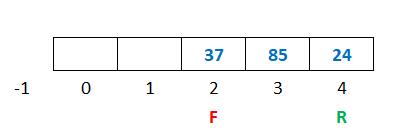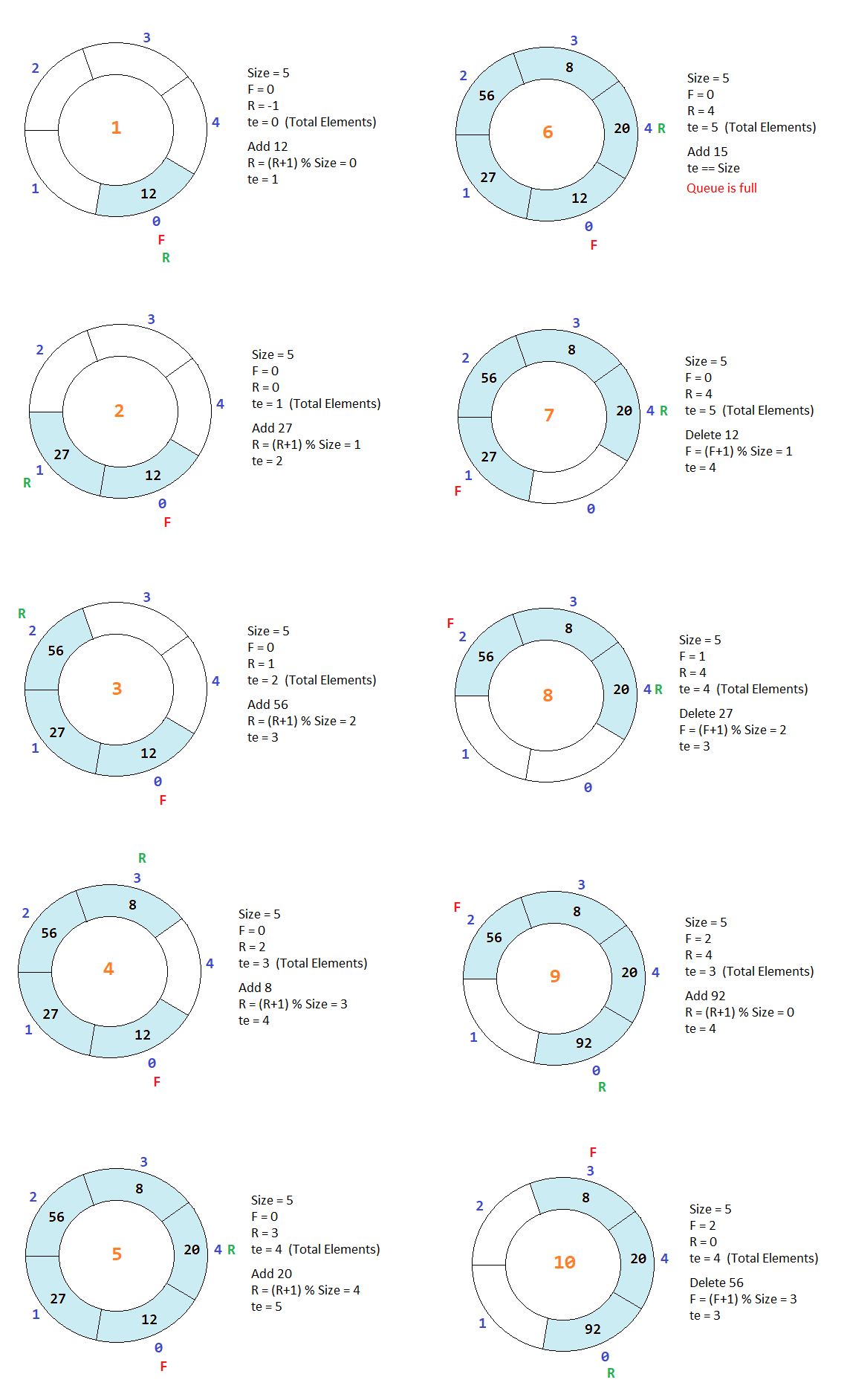# Circular Queue in Java Programming

###### Data Structure in Java

In this lesson, we will understand what is Circular Queue in Java Programming and how to create them along with some examples.

## What is Circular Queue in Java

A Circular Queue in Java is a data structure in which elements are stored in a circular manner. In Circular Queue, after the last element, the first element occurs.

A Circular Queue is used to overcome the limitation we face in the array implementation of a Queue. The problem is that when the rear reaches the end and if we delete some elements from the front and then try to add a new element in the queue, it says "Queue is full", but still there are spaces available in the queue. See the example given below.In the above image, the queue is full because the rear R reached the end of the queue. We have deleted two elements from the queue, so the front F is at index 2. We can see that there are spaces available in the queue, but we can't add a new element because the rear can't go back to index 0.## Operation on Circular Queue

There are two operations possible on the circular queue.

• Add - When we add an element in the circular queue.
• Delete - When we delete an element from the circular queue.

To understand how the above operations work on a circular queue. See the example given below.From the above image, we can see that when we add a new element in the circular queue, the variable R is increased by R=(R+1)%Size, and the new element is added at the new position of R and te is increased by 1. Similarly, when we delete an element from the circular queue, the variable F is increased by F=(F+1)%Size and te is decreased by 1.

### Add Operation in Circular Queue

For add operation in the circular queue first, we check if the value of te is equal to the value of size then, we will display a message Queue is full, else we will increase the value of R by R=(R+1)%Size and add the element in the array at the new location of R and then increased the value of te by 1.

#### Example

``````if(te==size)
{
System.out.println("Queue is full");
}
else
{
R=(R+1)%size;
arr[R]=new_item;
te=te+1;
}``````

### Delete Operation in Circular Queue

For delete operation in the circular queue first, we check if the value of te is 0 then, we will display a message Queue is empty, else we will display the deleted element on the screen and then increase the value of F by F=(F+1)%Size and then decrease the value of te by 1.

#### Example

``````if(te==0)
{
System.out.println("Queue is empty");
}
else
{
System.out.println("Element Deleted = " + arr[F]);
F=(F+1)%size;
te=te-1;
}``````

## Program of Circular Queue using Array

Below is the complete program of circular queue in Java using an array having size 5.

#### Circular Queue Program in Java using Array

``````import java.util.Scanner;

public class Example
{
public static void main(String args[])
{
int size=5;
int arr[]=new int[size],R=-1,F=0,te=0,ch,n,i,x;
Scanner sc=new Scanner(System.in);

for(;;)		// An infinite loop
{
System.out.println("2. Delete");
System.out.println("3. Display");
System.out.println("4. Exit");
System.out.print("Enter Choice: ");
ch=sc.nextInt();

switch(ch)
{
case 1:
if(te==size)
{
System.out.println("Queue is full");
}
else
{
System.out.print("Enter a number ");
n=sc.nextInt();
R=(R+1)%size;
arr[R]=n;
te=te+1;
}
break;

case 2:
if(te==0)
{
System.out.println("Queue is empty");
}
else
{
System.out.println("Number Deleted = " + arr[F]);
F=(F+1)%size;
te=te-1;
}
break;

case 3:
if(te==0)
{
System.out.println("Queue is empty");
}
else
{
x=F;
for(i=1; i<=te; i++)
{
System.out.print(arr[x] + " ");
x=(x+1)%size;
}
System.out.println();
}
break;

case 4:
System.exit(0);
break;

default:
System.out.println("Wrong Choice");
}
}
}
}``````